Next: Conservation Laws Up: Mathematical Models of Fluid Previous: Stress Tensor in a

# Viscosity

The significance of the parameter, appearing in the previous expression for the stress tensor, can be seen from the form taken by the relation (1.25) in the special case of simple shearing motion. With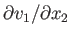as the only non-zero velocity derivative, all of the components of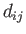are zero apart from the shear stresses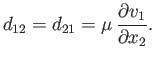(1.27)

Thus,is the constant of proportionality between the rate of shear and the tangential force per unit area when parallel plane layers of fluid slide over one another. This constant of proportionality is generally referred to as viscosity. It is a matter of experience that the force acting between layers of fluid undergoing relative sliding motion always tends to oppose the motion, which implies that.

The viscosities of dry air and pure water atand atmospheric pressure are about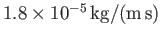and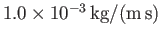, respectively. In neither case does the viscosity exhibit much variation with pressure. However, the viscosity of air increases by aboutpercent, and that of water decreases by about 3 percent, per degree Centigrade rise in temperature (Batchelor 2000).Next: Conservation Laws Up: Mathematical Models of Fluid Previous: Stress Tensor in a
Richard Fitzpatrick 2016-03-31